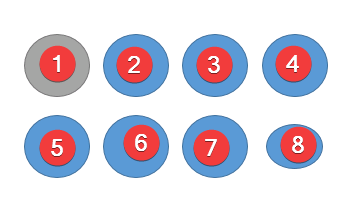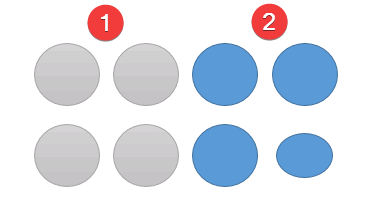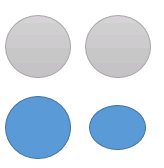# Divide and conquer algorithm

Divide and conquer algorithm divides problems into smaller subproblems , solve the subproblems and combine the solutions to find the solution of the main problem. Let’s see an example .

## Practical example

Suppose , you are given 8 balls , all balls are equal in weight except one ball. We’ll have to find the ball.Let’s follow brute force approach to find the solution. We’ll compare all other balls with the ball no 1 .

If any ball is less in weight than the first , then the ball is the desired ball. Now , how many comparison will need for this process ? There will be 7 comparison.

Let’s follow divide and conquer technique .

We can divide the balls in two parts and compare each other. Smaller ball is the one parts and that will be less in weight.Now again divide the other part in half and compare.We see blue part has less weight. So , again we divide and compare.Now , only two balls remaining. The ball is the less weight is the smaller ball we were searching for ! This process takes 4 comparison. So, this process has less complexity than the previous approach.

## Steps for Divide and conquer algorithm

• Divide: Divide the problem into smaller sub problems
• Conquer : Solve smaller problems.
• Combine : Combine the results from the sub problems to find the solution of actual problem.
Divide and conquer algorithms are generally recursive.

## Examples of Divide and conquer algorithm

• Binary Search
• Quick Sort
• Merge Sort
• Integer Multiplication
• Matrix Multiplication (Strassen’s algorithm)
• Maximal Subsequence

## Related posts